# Virtual Work and Static Equilibrium

• NovatoOfPhysica
In summary, the conversation discusses the use of virtual work in solving a problem involving the movement of blocks and weights. Two equations are presented, one involving a horizontal displacement and the other involving three different vertical displacements. The use of virtual work is questioned and the need for two equations is explained. The conversation ends with a request for a hint on how to continue with the problem.f

#### NovatoOfPhysica

Homework Statement
The system shown in Fig. 2-6 is in static equilibrium. Use the principle of
virtual work to find the weights A and B. Neglect the weight of the strings and
the friction in the pulleys.
Relevant Equations
Virtual Work Equations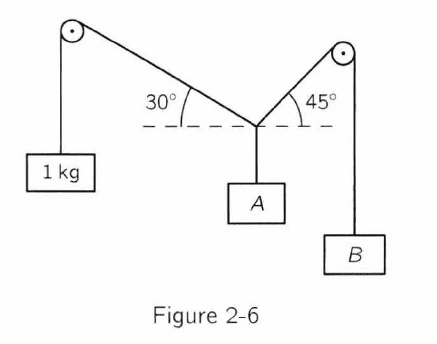Hi there, this question has already been discussed in this forum, however I do not know how to proceed further and if my reasoning is correct.

I start by imagining a downard displacement of the 1kg weight. As a consequence of this, block A moves upward and to the right. Also, block B moves upward at the same time. So I get the following formulas:

(1) dW = W1cos30dxcos0 + WBcos45dxcos180 = 0

(2) dW = W1sin30dh1cos0 + WAdhAcos180 + WBdhBcos180 = 0

(The last cos is the angle between the Force and the Displacement)

From the first equation I get the correct answer for WB. However, a couple of questions arise. First of all, isn't this form the same as the equation for the tension of both weights? That is, am I not using the principle of virtual work and instead am I using a conventional static method? Secondly, shouldn't there be only one virtual work equation instead of two or am I overthinking? (The last question comes because I watched a video where he could use dx and dy in only one equation if i recall correctly.)

From the second equation I do not know how to continue as I have three different variables dh1, dhA and dhB. What am I missing? Could you give me any hint so I can continue further?

PS I do not know how to format this question any tip and I'll try to edit it.

I start by imagining a downard displacement of the 1kg weight. As a consequence of this, block A moves upward and to the right. Also, block B moves upward at the same time. So I get the following formulas:

(1) dW = W1cos30dxcos0 + WBcos45dxcos180 = 0

(2) dW = W1sin30dh1cos0 + WAdhAcos180 + WBdhBcos180 = 0

(The last cos is the angle between the Force and the Displacement)

You can make your equations more readable if you use some of the tools available on the toolbar at the top of the posting window. For example: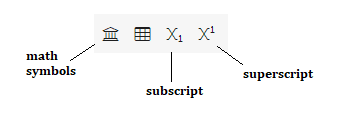Here is my attempt at reformating your equations:

(1) dW = W1 cos30 dx cos0 + WB cos45 dx cos180 = 0

(2) dW = W1 sin30 dh1 cos0 + WA dhA cos180 + WB dhB cos180 = 0

Please correct these if they do not represent what you intended.

Also, you should always define the symbols that you use. In particular, what do the quantities dx, dh1, dhA, and dhB represent?

Your second equation looks odd to me. Consider the first term on the right side. If dh1 is how far the 1 kg mass moves downward, then shouldn't the work done by gravity on this mass be W1⋅dh1⋅cos0 instead of W1⋅sin30⋅dh1⋅cos0? Why do you have a factor of sin30?

From the first equation I get the correct answer for WB. However, a couple of questions arise. First of all, isn't this form the same as the equation for the tension of both weights? That is, am I not using the principle of virtual work and instead am I using a conventional static method?
Feynman introduces the principle of virtual work in chapter 4 of volume 1 of the lectures. This is well before he discusses Newton's laws of motion in chapter 9. So this problem is probably meant to go with chapter 4. The principle of virtual work will yield the answer without having to use the idea of "net force equals zero for static equilibrium".

Secondly, shouldn't there be only one virtual work equation instead of two or am I overthinking? (The last question comes because I watched a video where he could use dx and dy in only one equation if i recall correctly.)
You need to be clear on what you are taking to be your virtual displacement(s) for the principle of virtual work. You have two unknowns (WA and WB). You will need two independent equations. You can get these by imagining two independent virtual displacements of the system. There are several possibilities. For example, if you want to use the principle of virtual work to get your equation (1), consider a virtual, horizontal displacement dx of the knot where the three strings meet.

From the second equation I do not know how to continue as I have three different variables dh1, dhA and dhB. What am I missing? Could you give me any hint so I can continue further?

For a particular virtual displacement of the system, the three displacements dh1, dhA, and dhB can be related by geometry and trig.

•NovatoOfPhysica and Delta2
You can make your equations more readable if you use some of the tools available on the toolbar at the top of the posting window. For example: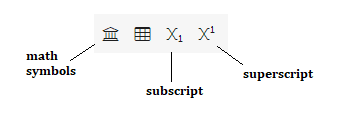Here is my attempt at reformating your equations:

(1) dW = W1 cos30 dx cos0 + WB cos45 dx cos180 = 0

(2) dW = W1 sin30 dh1 cos0 + WA dhA cos180 + WB dhB cos180 = 0

Please correct these if they do not represent what you intended.

Thank you for the tip, I'll use these tools from now on.

Yes, the equations are exactly what I wanted to write.

Also, you should always define the symbols that you use. In particular, what do the quantities dx, dh1, dhA, and dhB represent?

I had it on my paper (not posted) but I understand it would be clearer if I wrote what they were. So, dx represents a small displacement to the left, dh1 a small displacement downwards from W1, dhA a small displacement upwards from WA, and dhB a small displacement upwards from WB. I'll attach a picture so you can see my thought process better and point out any mistakes.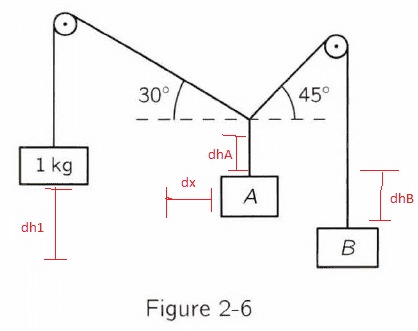All of these occur as a consequence of the small imaginary movement on W1.

Your second equation looks odd to me. Consider the first term on the right side. If dh1 is how far the 1 kg mass moves downward, then shouldn't the work done by gravity on this mass be W1⋅dh1⋅cos0 instead of W1⋅sin30⋅dh1⋅cos0? Why do you have a factor of sin30?

Hmm, the idea was similar to the problem 2.5 from the same book, Feynman Exercises.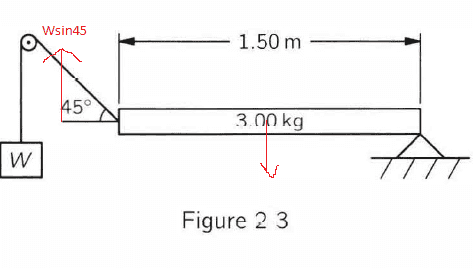As you can see, I had to decompose the tension into Wsin45 so I could take a small displacement dy. In this case, dy represents a y displacement from the left edge of the plank upwards.

But now that you pointed it out I can see, or at least think, of what could be the main differences. In the main question, we are considering dh1 as the displacement from W1 downwards and so the factor sin30 and sin45 should be written in WA because there is where the tensions occur. So, WA= W1sin30 + WBsin45. While in 2.5 (Fig 2.3) the tension occurs at the left edge and also the important displacement is in the edge and dh, the imaginary displacement of W, is not necessary.

Then the equation should become

(2) dW = W1 dh1 cos0 + WA dhA cos180 + WB dhB cos180 = 0

where WA = W1sin30 + WBsin45

I just saw that in the first (2) that I wrote, I didn't write WBsin45, following my wrong reasoning. I'd assume it was due to some conflict I was having between using the flawed equation or using the last version.

Feynman introduces the principle of virtual work in chapter 4 of volume 1 of the lectures. This is well before he discusses Newton's laws of motion in chapter 9. So this problem is probably meant to go with chapter 4. The principle of virtual work will yield the answer without having to use the idea of "net force equals zero for static equilibrium".

Indeed, I should use the principle of virtual work throughout this chapter. The main problem I have is from equation (1):

(1) dW = W1 cos30 dx cos0 + WB cos45 dx cos180 = 0

Cancelling dx and solving the cos we get

WB = √(3/2)

But from Newton's Laws:

W1cos30 = WBcos45

which is basically a reduced version of (1). So my question would be, am I applying virtual work on the worked solution provided above or am I going back to Newton's Laws?

You need to be clear on what you are taking to be your virtual displacement(s) for the principle of virtual work. You have two unknowns (WA and WB). You will need two independent equations. You can get these by imagining two independent virtual displacements of the system. There are several possibilities. For example, if you want to use the principle of virtual work to get your equation (1), consider a virtual, horizontal displacement dx of the knot where the three strings meet.

What do you mean by "two independent virtual displacements"? Is it what I've drawn in my picture? If not, could you please provide a picture so I can understand it better.

For a particular virtual displacement of the system, the three displacements dh1, dhA, and dhB can be related by geometry and trig.
Aha, I'll give it another try as I tried to relate them yesterday but I got a nonsensical answer. I'll come back to this post later. Could you correct any mistakes I made with my reasoning and if I am missing any important concept/idea as I have a hard time visualizing and solving problems with virtual work.

•Delta2
Hmm, the idea was similar to the problem 2.5 from the same book, Feynman Exercises.
View attachment 302798
As you can see, I had to decompose the tension into Wsin45 so ...

In applying the principle of virtual work, you don't need to think about the tension forces. All you need is the idea that for any infinitesimal virtual displacement of the system, the total work done by the external gravitational forces is zero. Or, as Feynman expresses it, "the sum of the heights times the weights doesn't change".

Indeed, I should use the principle of virtual work throughout this chapter. The main problem I have is from equation (1):

(1) dW = W1 cos30 dx cos0 + WB cos45 dx cos180 = 0

Cancelling dx and solving the cos we get

WB = √(3/2)

But from Newton's Laws:

W1cos30 = WBcos45

which is basically a reduced version of (1). So my question would be, am I applying virtual work on the worked solution provided above or am I going back to Newton's Laws?
You don't need Newton's laws. I don't know how you arrived at your equation (1). That is, I don't know if you got it from the principle of virtual work. I think you took the virtual displacement of the system to be a downward displacement of the 1 kg block by a distance dh1 with consequent horizontal and vertical displacements dxA and dhAof block A and a vertical displacement dhB of block B.

Now there is a peculiarity here. If all I know is that the 1 kg block moves downward by dh1, there is no way to determine the other displacements dxA, dhA, and dhB without making additional assumptions. To see this, note that I can make the 1 kg block move downward by displacing the knot only horizontally to the left by dxA so that dhA = 0. Or, I can make the 1 kg block move downward by displacing the knot only vertically by dhA so that dxA = 0. So, dxA and dhA are not determined by just knowing that the 1 kg block moves downward.

But this is actually to our advantage. This means that you can take the virtual displacement of the system to be the result of just a horizontal displacement of the knot by dxknot with no vertical displacement of the knot. Now you should be able to work out the displacements of the three blocks in terms of dxknot and set up the principle that the total gravitational work is zero. This will give you one equation to work with.

Next, you can take the virtual displacement of the system to correspond to a vertical displacement of the knot by dhknot with no horizontal displacement of the knot. The principle of virtual work will give you a second equation to work with.

What do you mean by "two independent virtual displacements"? Is it what I've drawn in my picture? If not, could you please provide a picture so I can understand it better.
The horizontal and vertical displacements of the knot are two independent virtual displacements.

You can imagine other possibilities. For example, you could have a virtual displacement of the system where the knot moves in such a direction that block B doesn't move at all. Or, you could have a virtual displacement such that block A doesn't move.

•NovatoOfPhysica and Delta2